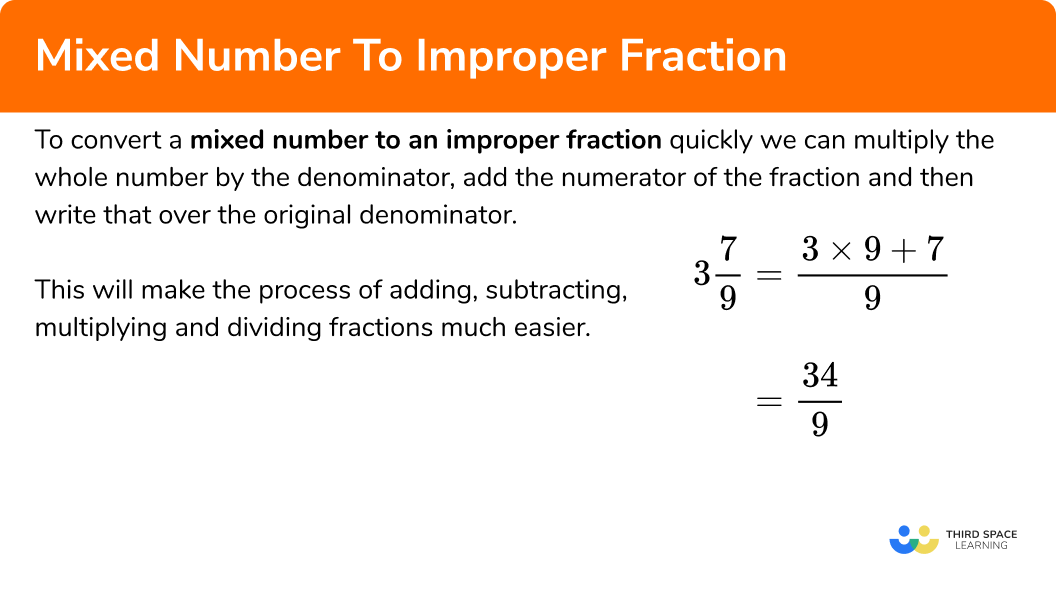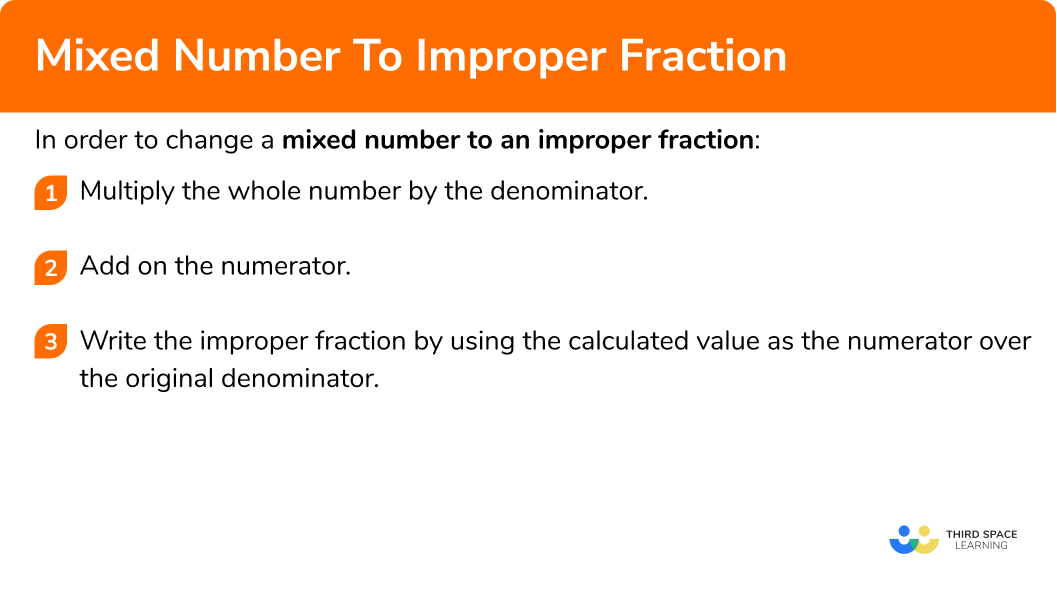GCSE Maths Number FDP Fractions

Mixed Number To Improper Fraction

# Mixed Number To Improper Fraction

Here we will learn about converting mixed numbers to improper fractions.

There are also improper fractions and mixed numbers worksheets based on Edexcel, AQA and OCR exam questions, along with further guidance on where to go next if you’re still stuck.

## What are mixed numbers and improper fractions?

A proper fraction has a numerator (the top number) that is smaller than the denominator (the bottom number) of the fraction.

An example of a proper fraction is \frac{8}{11} \

An improper fraction has a numerator that is bigger than the denominator of the fraction. Sometimes referred to as ‘top heavy’ fractions.

An example of an improper fraction is \frac{25}{7} \

A mixed number has a whole number part and a fractional part.

An example of a mixed number is 3 \frac{3}{4} \times 2 \frac{5}{8}

## What is the process for converting a mixed number to improper fraction?

Converting a mixed number to an improper fraction is an important skill. When calculating with mixed numbers, it can be much easier to perform the arithmetic required if the mixed numbers are converted to improper fractions.

A proper fraction has a numerator (the top number) that is smaller than the denominator (the bottom number) of the fraction.

An improper fraction has a numerator that is bigger than the denominator of the fraction. Sometimes referred to as ‘top heavy’ fractions.

For example,

If we needed to calculate 1 \frac{2}{3} \times 4 \frac{3}{5}, one way would be to use a grid method.

This can be a very long process involving lots of other fraction arithmetic. Converting the mixed numbers to improper fractions before multiplying is a way of simplifying the process.

To convert the mixed numbers to improper fractions we change the whole number to a fraction with the same denominator. We then add the numerators together and get a new numerator over the original denominator.

For example,

To convert a mixed number to an improper fraction quickly we can multiply the whole number by the denominator, add the numerator and then write that over the original denominator.

For example,

This will make the process of adding, subtracting, multiplying and dividing fractions simpler.

### What is converting mixed numbers to improper fractions?## How to convert a mixed number to improper fraction

In order to convert a mixed number to an improper fraction:

1. Multiply the whole number by the denominator.
3. Write the improper fraction by using the calculated value as the numerator over the original denominator.

### Explain how to convert mixed numbers to improper fractions### Related lessons on fractions

Mixed number to improper fraction is part of our series of lessons to support revision on fractions. You may find it helpful to start with the main fractions lesson for a summary of what to expect, or use the step by step guides below for further detail on individual topics. Other lessons in this series include:

## Converting mixed numbers to improper fractions examples

### Example 1: change a mixed number to an improper fraction

Write the following mixed number as an improper fraction, 2\frac{4}{5}.

1. Multiply the whole number by the denominator.

The whole number part of the mixed number is 2 and the denominator is 5, \ 2 \times 5 = 10.

The numerator is 4, \ 10 + 4 = 14.

The new numerator for the improper fraction is 14.

3Write the improper fraction by using the calculated value as the numerator over the original denominator.

2\frac{4}{5}=\frac{14}{5}

### Example 2: change a mixed number to an improper fraction

Write the following mixed number as a improper fraction, 3\frac{1}{6}.

The whole number part of the mixed number is 3 and the denominator is 6, \ 3 \times 6 = 18.

The numerator is 1, \ 18 + 1 = 19.

The new numerator for the improper fraction is 19.

3\frac{1}{6}=\frac{19}{6}

### Example 3: change a mixed number to an improper fraction

Write the following mixed number as a improper fraction, 4\frac{3}{7}.

The whole number part of the mixed number is 4 and the denominator is 7, \ 4 \times 7 = 28.

The numerator is 3, \ 28 + 3 = 31.

The new numerator for the improper fraction is 31.

4\frac{3}{7}=\frac{31}{7}

### Common misconceptions

A common error can occur when adding the whole number to the denominator instead of multiplying.

• Confusing mixed numbers and improper fractions

Mixed numbers are written with a whole number and a fractional part.

For example, 4 \frac{5}{6}.

Improper fractions are not written with a whole number part, but the numerator is always bigger than the denominator.

For example, \frac{29}{6}.

### Practice converting mixed numbers to improper fractions questions

1. Write the following mixed number as an improper fraction, 2\frac{3}{5}.

3\frac{2}{5}\frac{13}{5}\frac{23}{5}2\frac{1}{5}2\frac{3}{5}=\frac{2\times5+3}{5}=\frac{10+3}{5}=\frac{13}{5}

2. Write the following mixed number as an improper fraction, 5\frac{2}{7}.

\frac{37}{7}\frac{52}{7}\frac{25}{7}\frac{34}{7}5\frac{2}{7}=\frac{5\times7+2}{7}=\frac{35+2}{7}=\frac{37}{7}

3. Write the following mixed number as an improper fraction, 8\frac{1}{4}.

\frac{81}{4}\frac{33}{8}\frac{35}{4}\frac{33}{4}8\frac{1}{4}=\frac{8\times4+1}{4}=\frac{32+1}{4}=\frac{33}{4}

4. Write the following mixed number as an improper fraction, 5\frac{3}{11}.

\frac{16}{11}\frac{55}{11}\frac{58}{11}\frac{58}{3}5\frac{3}{11}=\frac{5\times11+3}{11}=\frac{55+3}{11}=\frac{58}{11}

5. Write the following mixed number as an improper fraction, 4\frac{2}{13}.

\frac{21}{13}\frac{54}{13}\frac{19}{13}\frac{52}{13}4\frac{2}{13}=\frac{4\times13+2}{13}=\frac{52+2}{13}=\frac{54}{13}

6. Write the following mixed number as an improper fraction, 2\frac{8}{9}.

\frac{26}{9}\frac{25}{9}\frac{19}{9}\frac{16}{9}2\frac{8}{9}=\frac{2\times9+8}{9}=\frac{18+8}{9}=\frac{26}{9}

### Converting mixed numbers to improper fractions GCSE questions

1. Choose the fraction which is the same as 5 \frac{3}{7}?

\frac{22}{7} \hspace{1cm} \frac{38}{7} \hspace{1cm} \frac{15}{7} \hspace{1cm} \frac{35}{7}

(1 mark)

\frac{38}{7}

(1)

2. (a) Write 3.2 as an improper fraction in its simplest form.

(b) Work out 3\frac{1}{4}-1\frac{1}{3}.

(5 marks)

(a)

For any correct mixed number. For example, 3\frac{2}{10}.

(1)

\frac{16}{5}

(1)

(b)

Converting one of the fractions to an improper fraction. For example, \frac{13}{4} or \frac{4}{3}.

(1)

Write both fractions with a common denominator. For example, \frac{39}{12}, \frac{16}{12}.

(1)

\frac{23}{12} or 1 \frac{11}{12}.

(1)

3. Work out 4 \frac{1}{2} \div 3\frac{2}{3}.

(3 marks)

Converting one of the fractions to an improper fraction. For example, \frac{9}{2} or \frac{11}{3}.

(1)

Correct process to divide used. For example, finding common denominator for multiplying by the reciprocal.

(1)

\frac{27}{22} or 1 \frac{5}{22}.

(1)

## Learning checklist

You have now learned how to:

• Convert a mixed number to an improper fraction

## Still stuck?

Prepare your KS4 students for maths GCSEs success with Third Space Learning. Weekly online one to one GCSE maths revision lessons delivered by expert maths tutors.

Find out more about our GCSE maths tuition programme.